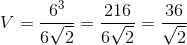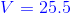## Example Questions

### Example Question #13 : Volume

Find the volume of a regular tetrahedron if one of its edges is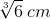long.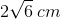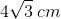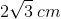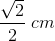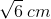Explanation:

Write the volume equation for a tetrahedron.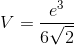In this formula,stands for the tetrahedron's volume and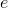stands for the length of one of its edges.

Substitute the given edge length and solve.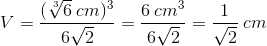Rationalize the denominator.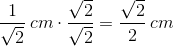### Example Question #14 : Volume

Find the volume of a tetrahedron if the side length is.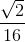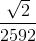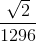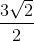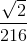Explanation:

Write the equation to find the volume of a tetrahedron.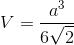Substitute the side length and solve for the volume.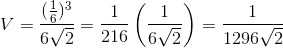Rationalize the denominator.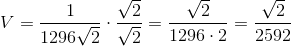### Example Question #11 : How To Find The Volume Of A Tetrahedron

What is the volume of a regular tetrahedron with an edge length of 6?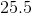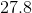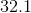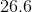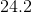Explanation:

The volume of a tetrahedron can be solved for by using the equation:whereis the measurement of the edge of the tetrahedron.

This problem can be quickly solved by substituting 6 in for